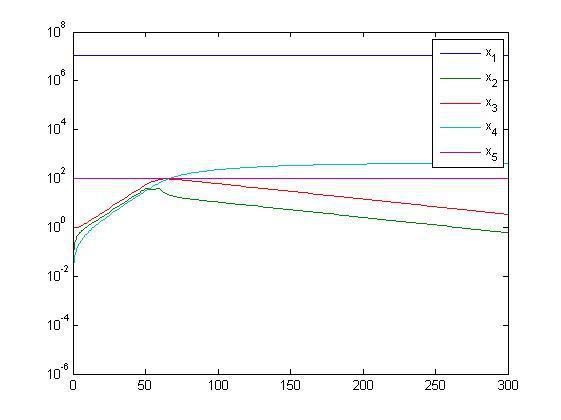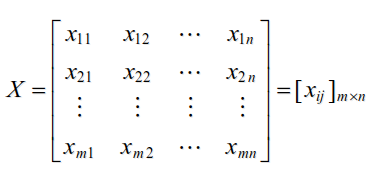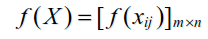• 51时你没给a赋值, 所以我把t==51改成了t<=51了, 这样的代码如下:f=@(t,x)[-3*10^(-8)*(1-((t<=51)*0.4+(((t<=60)&(t>51))*0.7)+(t>60)*0.9))*x(3)*x(1)-1*10^(-11)*(1-((t<=51)*0.4+(((...
匿名用户1级2015-02-07 回答因为t<51时你没给a赋值, 所以我把t==51改成了t<=51了, 这样的代码如下:f=@(t,x)[-3*10^(-8)*(1-((t<=51)*0.4+(((t<=60)&(t>51))*0.7)+(t> 60)*0.9))*x(3)*x(1)-1*10^(-11)*(1-((t<=51)*0.4+(((t<=60)&(t>51))*0.7)+(t> 60)*0.9))*x(5)*x(1)-1*10^(-11)*((t<=51)*0.4+(((t<=60)&(t>51))*0.7)+(t> 60)*0.9)*0.05*x(1)*x(5);3*10^(-8)*x(3)*x(1)*(1-((t<=51)*0.4+(((t<=60)&(t>51))*0.7)+(t> 60)*0.9))+3*10^(-8)*(1-((t<=51)*0.4+(((t<=60)&(t>51))*0.7)+(t> 60)*0.9))*(1-((t<=51)*0.4+(((t<=60)&(t>51))*0.7)+(t> 60)*0.9))*x(5)*x(3)+3*10^(-8)*(1-((t<=51)*0.4+(((t<=60)&(t>51))*0.7)+(t> 60)*0.9))*x(3)*((t<=51)*0.4+(((t<=60)&(t>51))*0.7)+(t> 60)*0.9)*0.05*x(5)-0.2*x(2);0.2*x(2)-0.05*x(3);0.05*x(3);1*10^(-11)*(1-((t<=51)*0.4+(((t<=60)&(t>51))*0.7)+(t> 60)*0.9))*x(5)*x(1)+1*10^(-11)*x(5)*((t<=51)*0.4+(((t<=60)&(t>51))*0.7)+(t> 60)*0.9)*0.05*x(1)-3*10^(-8)*x(3)*(1-((t<=51)*0.4+(((t<=60)&(t>51))*0.7)+(t> 60)*0.9))*(1-((t<=51)*0.4+(((t<=60)&(t>51))*0.7)+(t> 60)*0.9))*x(5)-3*10^(-8)*x(3)*(1-((t<=51)*0.4+(((t<=60)&(t>51))*0.7)+(t> 60)*0.9))*((t<=51)*0.4+(((t<=60)&(t>51))*0.7)+(t> 60)*0.9)*0.05*x(5)];t_final=300;x0=[11000000;0;1;0;100];[t,x]=ode45(f,[0,t_final],x0);semilogy(t,x)  % 这样y轴好看点.legend x_1 x_2 x_3 x_4 x_5如果使用函数文件, 应该新编函数f.m如下:function y=f(t,x)if t<=51   % 这里我改了.a=0.4;end;if (t<=60)&(t>51)a =0.7;end;if t>60a=0.9;end;y=[-3*10^(-8)*(1-a)*x(3)*x(1)-1*10^(-11)*(1-a)*x(5)*x(1)-1*10^(-11)*a*0.05*x(1)*x(5);3*10^(-8)*x(3)*x(1)*(1-a)+3*10^(-8)*(1-a)*(1-a)*x(5)*x(3)+3*10^(-8)*(1-a)*x(3)*a*0.05*x(5)-0.2*x(2);0.2*x(2)-0.05*x(3);0.05*x(3);1*10^(-11)*(1-a)*x(5)*x(1)+1*10^(-11)*x(5)*a*0.05*x(1)-3*10^(-8)*x(3)*(1-a)*(1-a)*x(5)-3*10^(-8)*x(3)*(1-a)*a*0.05*x(5)];end这时主程序代码是:t_final=300;x0=[11000000;0;1;0;100];[t,x]=ode45(@f,[0,t_final],x0);semilogy(t,x)legend x_1 x_2 x_3 x_4 x_5结果和刚才的是一样的.
展开全文• ; margin-right:0pt">t=((2*h-H^2)*sqrt(v2^2-v^2))/v*v2+delta/v2 ; margin-right:0pt">上边是需要构造的方程，一共六个变量，已知t，H,v,delta；求解v2,h（...有问必答
• 2.矩阵的寻访与赋值在创建了矩阵之后，我们经常需要访问矩阵中的某一个或者一些元素，另外可能需要对其中的某些元素重新赋值或者删除某一部分元素。本节介绍如何进行矩阵的寻访与赋值。2.1矩阵的标识本小节介绍单个...
2.矩阵的寻访与赋值在创建了矩阵之后，我们经常需要访问矩阵中的某一个或者一些元素，另外可能需要对其中的某些元素重新赋值或者删除某一部分元素。本节介绍如何进行矩阵的寻访与赋值。2.1 矩阵的标识本小节介绍单个元素标识和寻访的3种方式：全下标、单下标、逻辑1标识。1．全下标标识经典数学教科书在引述具体矩阵元素时，通常采用全下标标识法，即指出某一元素是在第几行第几列。这种标识方法的优点是：几何概念清楚，引述简单。全下标标识法在MATLAB的寻访和赋值中因为最为直观，所以它最为常用。对于二维矩阵来说，全下标标识由两个下标组成：行下标、列下标。如A(3,5)表示二维矩阵A的第3行第5列。这里值得注意的是，MATLAB中对下标的标识是从1开始的，就是和我们平时在数学中使用的说法是一致的。这和其他一些编程语言从0开始标识是不同的。2．单下标标识MATLAB尽管是以矩阵作为基本的计算单元，但是矩阵的后台存储并不是像显示出来的那样成长方形排列的，而是按照单下标标识作为一列存储到内存中。单下标标识就是“只用一个下标来指明元素在矩阵中的位置”。当然，这样做首先要对二维矩阵的所有元素进行“一维编号”。所谓“一维编号”就是：先设想把二维矩阵的所有列，按照先左后右的次序首尾相连排成一维长列，然后自上而下对元素位置进行编号。单下标与全下标的转换关系：以m´n的二维矩阵A为例，若全下标的元素位置是“第a行，第b列”，那么相应的单下标则为c=(b-1)*m+a。在MATLAB中，有两个函数可以实现全下标和单下标的转换。sub2ind：    根据全下标换算出单下标。ind2sub：    根据单下标换算出全下标。单下标的优势是在特定情境下使用更为简洁，例如编制某些循环的时候只需要一个循环变量就可以了，另外比如需要将某数组赋值给另一维数不同的数组的时候。3．逻辑1标识在实际使用中，有时会遇到寻找矩阵中大于或者小于某值的元素的问题，这时就可以使用逻辑1标识法。逻辑1标识用一个基于原矩阵A相对位置的逻辑数组B来对矩阵A进行寻访。数据B中每一个true值也就是1表示相对位置的A中元素可以被寻访。如果需要通过逻辑1标识来对矩阵进行寻访，只需将符合条件的元素位置的标识设置为逻辑1即可。采用逻辑1标识的程序在速度方面具有一定的优势。2.2 矩阵的寻访【例2-7】 二维矩阵的寻址。>> a=[1 2 3; 4 5 6]          %  创建测试矩阵1     2    34     5    6>> A=a(2,2)            %  全下标寻访>> b=a(4)              %  单下标寻访>> B=a>5                    %  返回逻辑下标0     0    00     0    1>> c=a(B)              %  逻辑下标寻访>> d=a(1,:)             %  通过使用冒号可以寻访全行元素1     2    3>> e=a(:,2)             %  通过使用冒号可以寻访全列元素>> f=a(:)               %  单下标寻访>> g=a(:,[1 3])              %  寻访地址可以是向量，以同时寻访多个元素1     34     6本例中的B=a>5和c=a(B)，就是采用逻辑1标识法访问矩阵a中大于5的元素。2.3  矩阵的赋值在了解了矩阵的寻访方法以后，给矩阵中的特定元素赋值也就成了一个很简单的事情。下面举例来说明。【例2-8】 二维矩阵的赋值。>> a=magic(4)16     2    3    135    11   10     89     7    6    124    14   15     1>> a(3,4)=0    % 对单个元素进行赋值16     2    3    135    11   10     89     7    6     04    14   15     1>> a(:,1)=1   % 对第一列进行赋值1     2    3    131    11   10     81     7    6     01    14   15     1>> a(14)=16       % 采用全下标对第14个元素进行赋值1     2    3    131   11    10     161     7    6     01    14   15    12.3.1 进行数组运算的常用函数在MATLAB中有一些常用函数，这些函数在日常的编程计算过程中会经常遇到，一般是基本的数学概念在MATLAB中的函数表达方式。这些函数在MATLAB中可以同时作用于整个矩阵或者数组，应用起来非常方便，不需要再另写循环程序来对各元素分别进行计算。掌握这些函数是进一步学习的基础。MATLAB人性化的地方在于其自带函数基本是按照相对应的英文名称缩写而来，所以便于记忆。2.3 函数数组运算规则的定义对于(m´n)的数组，函数的数组运算规则是指：也就是说函数的数组运算是指将函数作用于矩阵中的每一个元素，并将最后的结果储存为与原矩阵行列数相同的矩阵。2.3.2 进行数组运算的常用函数本小节列出进行数组运算的常用函数。常用基本数学函数见表2-2，常用三角函数见表2-3，常用适用于向量的函数见表2-4。表2-2         MATLAB常用的基本数学函数函    数说    明函    数说    明纯量的绝对值或向量的长度将实数x化为分数表示复数z的相角符号函数 当x<0时，sign(x)=-1； 当x=0时，sign(x)=0;  当x>0时，sign(x)=1求x除以y的余数复数z的实部整数x和y的最大公因数复数z的虚部整数x和y的最小公倍数复数z的共轭复数自然指数四舍五入至最近整数无论正负，向0的方向取最近整数以e为底的对数，即自然对数舍去法取最近整数以2为底的对数进一法取最近整数以10为底的对数表2-3        MATLAB常用的三角函数函     数说     明函     数说     明正弦函数超越正弦函数余弦函数超越余弦函数正切函数超越正切函数反正弦函数反超越正弦函数反余弦函数反超越余弦函数反正切函数反超越正切函数四象限的反正切函数表2-4        适用于向量的常用函数函     数说     明函     数说     明向量x的元素的最小值向量x的欧氏长度，也就是范数向量x的元素的最大值向量x的元素总和向量x的元素的平均值向量x的元素总乘积向量x的元素的中位数向量x的累计元素总和向量x的元素的标准差向量x的累计元素总乘积向量x的相邻元素的差向量x和y的内积对向量x的元素进行排序cross(x, y)向量x和y的外积【例2-9】  数组运算示例。1     2    4     916    25   36    49>> b=sqrt(a)                   %  应用函数对矩阵中的每一个元素分别开方
展开全文• 背景：基于稀疏表示的图像融合时，两幅图像通过比较各自稀疏系数的最大值来得到融合后的稀疏系数，之后再重建融合图像 ... % 得到稀疏矩阵非零的项，包括横纵坐标和对应数值 [row,col]=size(aa1); aa3=sparse(row,c
背景：基于稀疏表示的图像融合时，两幅图像通过比较各自稀疏系数的最大值来得到融合后的稀疏系数，之后再重建融合图像
稀疏融合时：
a1=[0 1 0;
2 0 1;
1 0 1]
a2=[2 0 0;
0 0 3;
0 2 4];
aa1=sparse(a1);			% 得到稀疏矩阵
aa2=sparse(a2);
c1=find(aa1);			% 得到稀疏矩阵非零的项，包括横纵坐标和对应数值

[row,col]=size(aa1);
aa3=sparse(row,col);	% 构建新的稀疏矩阵

for i=c1				% 按照非零项的位置赋值，得到的aa3是和aa1一样的矩阵
aa3(i)=aa1(i);
end
[x,y,z]=find(aa2);		% x为非零项的横轴值，y为纵轴值，z为（x，y）点的数值

for m=1:length(x)
if(aa2(x(m),y(m))>aa1(x(m),y(m)))
aa3(x(m),y(m))=aa2(x(m),y(m));		% 比较得到最大值，赋值到相应的坐标值位置点
end
end

运行结果
>> aa1

aa1 =

(2,1)        2
(3,1)        1
(1,2)        1
(2,3)        1
(3,3)        1

>> aa2

aa2 =

(1,1)        2
(3,2)        2
(2,3)        3
(3,3)        4
>> aa3

aa3 =

(1,1)        2
(2,1)        2
(3,1)        1
(1,2)        1
(3,2)        2
(2,3)        3
(3,3)        4



展开全文机器学习
• B(1:3, 2:4) = A %将A赋值到B的第1行到3行，第2列岛4列，注意维度要匹配 或 B(1:2, 2:3) = A（2:3, 1:2） %将A的部分内容赋值给B的指定位置 个人觉得这比单个元素的赋值方便多了，减少for循环的使用 转载于:ht...
目前还没见到网上用过这个简单的方式
A=
[1 2 3; 4 5 6; 7 8 9]
B =
zeros(5,5)
B(1:3, 2:4) = A     %将A赋值到B的第1行到3行，第2列岛4列，注意维度要匹配
或
B(1:2, 2:3) = A（2:3, 1:2）   %将A的部分内容赋值给B的指定位置
个人觉得这比单个元素的赋值方便多了，减少for循环的使用
转载于:https://www.cnblogs.com/niulang/p/9143435.html
展开全文• MATLAB里边定义 a = [1, 4, 2; 3, 2, 0]; 在C语言等效定义是 double a = { 1, 3, 4, 2, 2, 0 };//c语言的数组在内存里边是按行依次存储的，而MATLAB的mxArray是按列依次存储...//定义一个2行3列的矩阵 me...
• 由此可以创建很大的矩阵空间，而由于在计算的时候矩阵数据存在几十万和上百万的情况，如果矩阵中非0数据占总矩阵大小的5%以下，可以使用稀疏矩阵方式优化矩阵空间或矩阵分块存储，下面使用matlab如何创建稀疏矩阵并...
• matlab字符串判断每个字符，空矩阵赋值 ** 下面是题目 首先，注意 == 的两个用法 字符串与单个字符比较 字符串与同维字符串比较 这道题困扰我的点就是 我想把不一样的字符存进一个矩阵中，但是不知道怎么创建一...
• matlab syms 这是一个针对于matlab 中 syms 矩阵赋值的示例。
• 现象：嵌套两层for循环，对图像的行列依次点对点赋值给另一个矩阵，只有w个数据成功赋值，其余全为0。 这里并不是无聊而是确实有场景需要用到点对点的赋值+运算。 现象1（单个例子） 我是严重怀疑我用了一个假的...
• *内容摘要 ：该代码用于实现在MATLAB矩阵及元素的赋值 *文件标识：无 *作 者：*完成日期：2019-3-10 *问题描述：给矩阵a赋值 >> a=[1 4 7;2 5 8; 3 6 9] a = 1 4 7 2 5 8 3 6 9 *问题描述...
• Matlab 矩阵学习笔记 # 阅读本篇文章你可以学习到： - Ⅰ Matlab矩阵或数组的表示 - Ⅱ 矩阵的分类 - Ⅲ 矩阵如何输入如何生成 - Ⅳ 矩阵元素下标 - Ⅴ 子矩阵块的产生方法 - Ⅵ 矩阵的赋值方法
• MATLAB:创建一个矩阵，大小为12，并对矩阵赋值，每行都是1到12；用matlab自带的sum函数对矩阵列求和、行求和、总和。 1、两个for循环： a=zeros(12); [i,j]=size(a); for i=1:12; for j=1:12; a(i,j)=i,j end end 一...
• 2.熟练掌握matlab 的变量、矩阵定义和基本赋值符号；3.熟练掌握matlab 矩阵的引用及矩阵初等运算实验内容：1. 定义矩阵[2.3,5.8,9]a =；2. 计算a 的正弦值；3. 对a 中每个元素加3；4. 定义矩阵[5.2,3.14,2]b =；5. a...
• 今天看代码时，遇到了一个令我有点惊讶的一个赋值问题。 虽然是一个很小很小的问题，很多人可能都不会...%a(10:13) %若不赋值，只是通过下标访问数组 则会出现错误：索引超出矩阵维度。 %%%%上边所示都是一维数组 b=[1
• 两个系数矩阵要进行融合，采用绝对值取大的准则，一般采用for循环即可 Coefs=Coefs1; [x,y,z]=find(Coefs2); for m=1:length(x) if(abs(Coefs2(x(m),y(m)))>abs(Coefs1(x(m),y(m)))) Coefs(x(m),y(m))=...
• fileFolder=fullfile('D:\MATLAB\bin\trc'); dirOutput=dir(fullfile(fileFolder,'*.trc')); fileNames={dirOutput.name}; 解释： 1、第一行 fileFolder=fullfile('D:\MATLAB\bin\trc')...
• Matlab常用矩阵操作： 1.求矩阵A的逆矩阵： inv(A) 2.求特征值、特征向量： [a,b]=eig(A) %同时求特征值、特征向量 a=eig(A) %只求特征值 3.在矩阵中使用变量（未知数）： syms a %表示定义了一个变量a，...
• 第2章 MATLAB矩阵及其运算;数据术语 矩阵由mn个数组成的排成m行n列的一个矩形的数表其中00矩阵为空矩阵([ ])数表中第i(1im)行第j(1jn)列的数据称为矩阵元素 标量1 1向量行向量(1 n)列向量是矩阵的特例;2.1 变量和...
• 1.矩阵赋值 a=1:5 %产生1行5列的行向量 a=[1:5]’ %产生5行1列的列向量 a= [ 1 2 3;4 5 6;7 8 9] a =[… 1 2 3 4 5 6 7 8 9] 2.文本中导入数据 如存在文本文件data.txt文件内容如下： 20020101 31 25 30 2...
• 计算物理基础 主讲马堃 计算物理基础 第2章Matlab矩阵及其运算 2.1 变量和数据操作 2.2 Matlab 矩阵 2.3 Matlab 运算 2.4 矩阵分析 2.5 矩阵的超越函数 2.6 字符串 2.7 结构数据和单元数据 计算物理基础 2.1 变量和...
• 1.直接赋值 [ ] %开始结束标志 ；或按enter %行与行之间分隔开 ，或空格 %元素之间的分开 >> A = [1 2 3;4 5 6;7 8 9] A = 1 2 3 4 5 6 7 8 9 2.冒号表达式 e1:e2:e3 %e1为初始值，e2为步长，e3为...matlab
• 使用c++实现Matlab矩阵Matrix类，是今后c++实现Matlab函数功能的基础，保留了Matlab中矩阵复制、赋值，加减乘数算数运算，()运算，正负号运算，以及矩阵使用类似Matlab中的生成方式，子矩阵读取，矩阵的扩充，变换等...matrix c++
• 1.MATLAB赋值语句 （1）变量=表达式 （2）表达式 其中表达式是用运算符将有关运算量连接起来的式子...2.MATLAB矩阵 （2.1）矩阵的建立 直接输入法：最简单的建立矩阵的方法是从键盘直接输入矩阵的元素。具体方...MATLAB基础学习 MATLAB基础教程 MATLAB m文件 MATLAB特殊矩阵...

# matlab矩阵赋值matlab 订阅